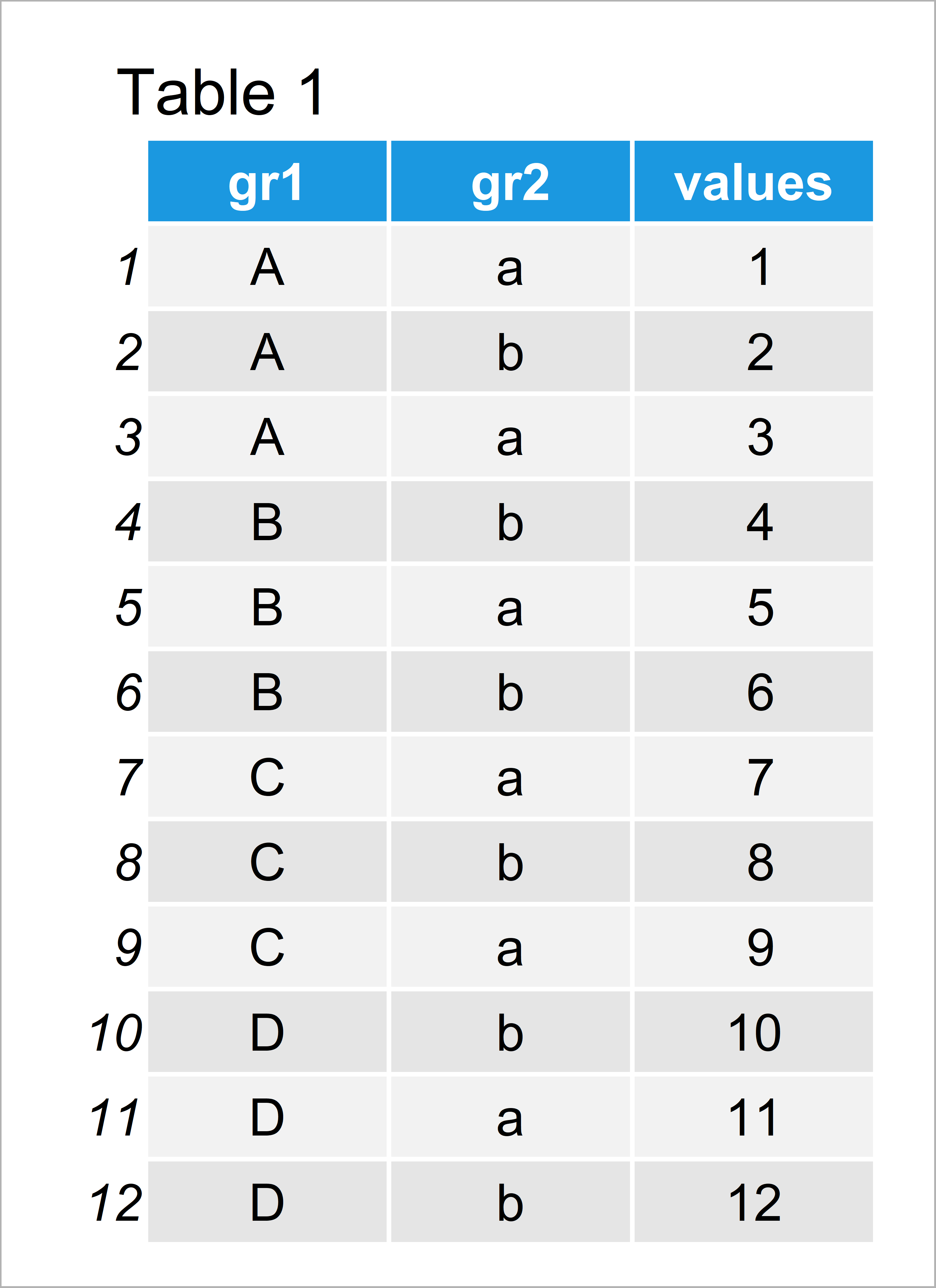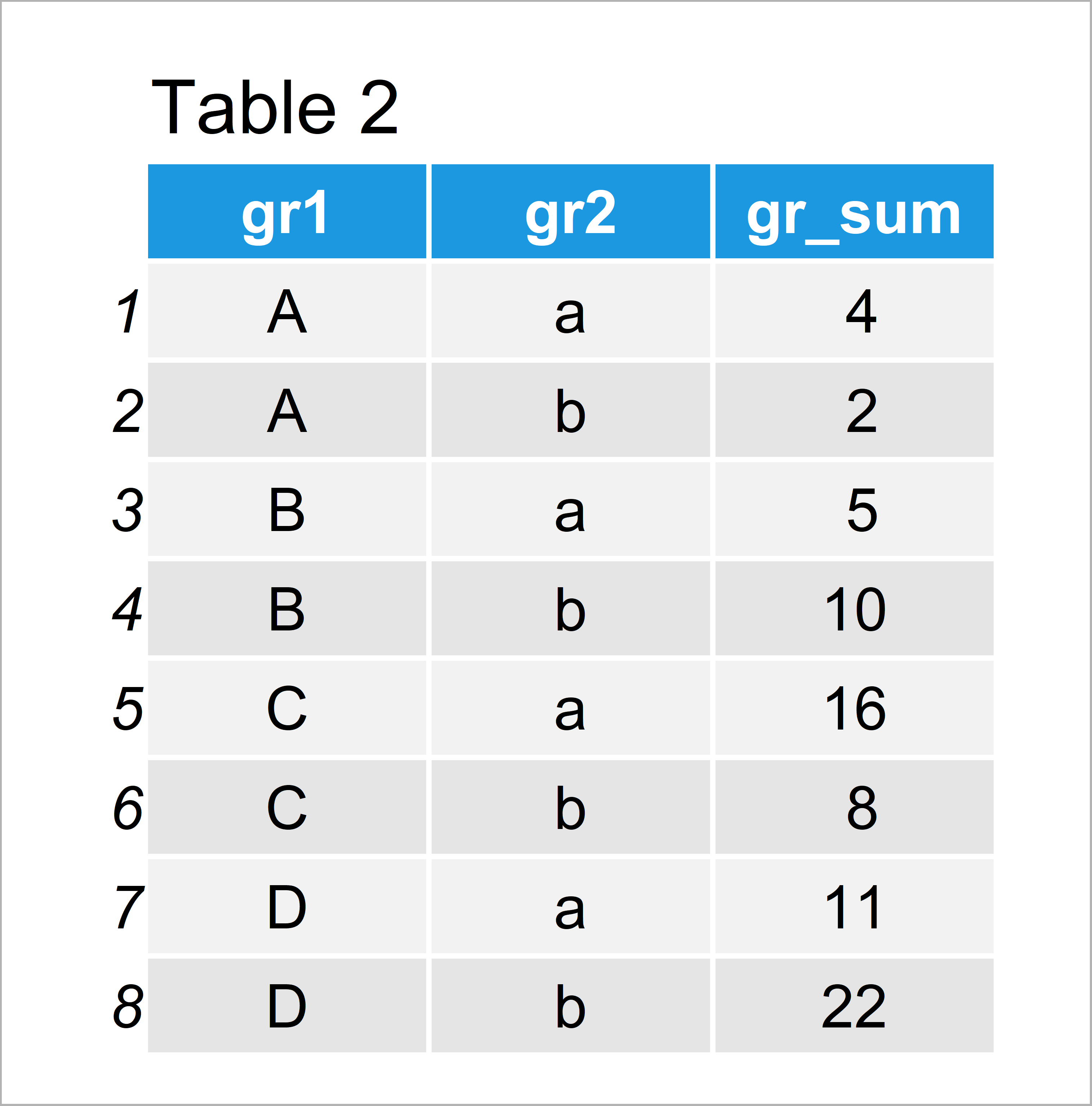# Group Data Frame by Multiple Columns in R (Example)

This article explains how to group a data frame based on two variables in R programming.

The article is structured as follows:

Here’s the step-by-step process:

## Construction of Example Data

Have a look at the example data below:

```data <- data.frame(gr1 = rep(LETTERS[1:4], each = 3),  # Create example data
gr2 = letters[1:2],
values = 1:12)
data                                                   # Print example data```As you can see based on Table 1, our example data is a data frame consisting of twelve data points and the three columns “gr1”, “gr2”, and “values”.

## Example: Group Data Frame Based On Multiple Columns Using dplyr Package

This example explains how to group and summarize our data frame according to two variables using the functions of the dplyr package.

In order to use the functions of the dplyr package, we first have to install and load dplyr:

```install.packages("dplyr")                              # Install & load dplyr package
library("dplyr")```

Next, we can use the group_by and summarize functions to group our data. In order to group our data based on multiple columns, we have to specify all grouping columns within the group_by function:

```data_group <- data %>%                                 # Group data
group_by(gr1, gr2) %>%
dplyr::summarize(gr_sum = sum(values)) %>%
as.data.frame()
data_group                                             # Print grouped data```By executing the previous R code we have created Table 2, i.e. a data frame that has been grouped by two variables.

Note that we have calculated the sum of each group. However, it would also be possible to compute other descriptive statistics such as the mean or the variance.

Also, note that we have converted our final output from the tibble to the data.frame class. In case you prefer to work with tibbles, you may remove the last line of the previous R code.

## Video & Further Resources

Would you like to know more about the grouping of data frames? Then you might watch the following video of my YouTube channel. In the video, I show the R programming syntax of this tutorial:

In addition, you might want to read the related tutorials of my website.

To summarize: This tutorial has demonstrated how to group a data set by multiple columns in R. If you have additional questions, please let me know in the comments below.

Subscribe to the Statistics Globe Newsletter